# Integral of sec(x)

This integral needs a quite a bit of work but we will eventually be able to evaluate it! Keep in mind that there is a much faster method, but it’s not intuitive at all. In this post I want to show you the method I have come up with and, honestly, it is much more interesting. With this being said, keep reading to learn how to integrate the trigonometric function sec(x). But first,

What is sec(x)?

In order to solve this integral, we need to write sec(x) in another form, i.e. in terms of cos(x). Well, after all, the secant is the reciprocal of the cosine: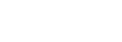Integral of sec(x)Red: graph of the function f(x)=sec(x); blue: graph of the primitive F(x)=ln(sec(x)+tan(x)).

Now we can write the integral in terms of cos(x):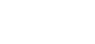What can we do? I really don’t think u-substitution will work, but let’s try anyway: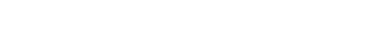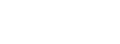We are integrating with respect to u, so x‘s are not accepted. Notice that we have sin(x) at the denominator, and we know that u=cos(x); this means x=arccos(u). I can already tell you that this is not going to turn out nicely.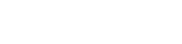Trust me, this is not good.

Let’s try performing integration by parts: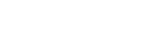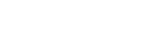So what can we do? Well, there actually is something we can do. “1”, what is “1”? Remember the most important trigonometric identity?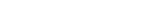And this is what we need to know!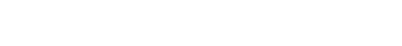This is so much better! Let’s now simplify things a little: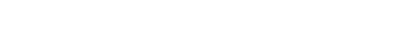Let’s apply linearity: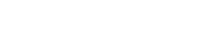The integral of cos(x) is sin(x):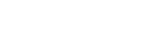Let’s now solve this integral. Notice that cos(x) is equal to the square root of cos²(x) and, from the identity sin²(x)+cos²(x), we know that cos²(x) is equal to 1-sin²(x). This means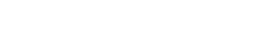At this point, what I though was to let u=sin(x); this way we would have: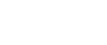Now something interesting: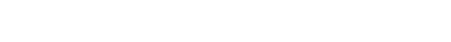We said that cos(x) is equal to the square root of 1-sin²(x), but we also let u=sin(x), hence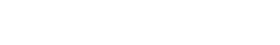This step is necessary, since we are integrating with respect to u.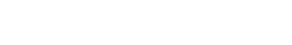This is so much better than the original integral, mostly because there are no trigonometric functions. Obviously, this doesn’t always mean that the integral is an easy one.

The first things that pops to the eye is 1-u², which is a difference of squares.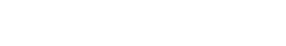At this point, let me introduce you another method of integration. It’s not really “of integration”, but it’s very helpful when it comes to products of fractions under the integral sign. It’s called partial fraction decomposition.

Partial fraction decomposition

A fraction of this type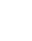where f and g are first degree polynomial functions of the variable of integration x, can be written as a sum of two fractions: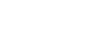Take the lowest common multiple and you get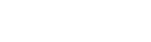If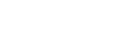then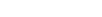We said that f and g are first degree polynomial functions, i.e.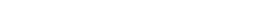Therefore,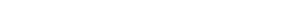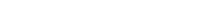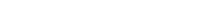Factor out the x‘s: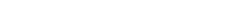This can be written as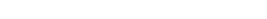The coefficient of the x on the left side is equal to the coefficient of the x on the right side, and the constant Ad+Bb is equal to the constant on the right side, here 1.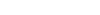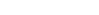This is a system of two equations, where the unknowns are A and B. After using substitution, we get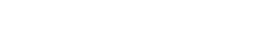At this point, you plug in a, b, c and d values and you find A and B, which you then substitute into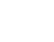and you have a sum of two fractions. I shoud mention that if the numerator is, for example, 2, it’s as if the fraction with 1 as numerator was multiplied by 2. The same happens for anything at the numerator.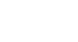Let’s decompose our fraction: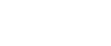As we’ve just said, we can write this as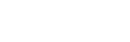Great! Now, let’s perform partial fraction decomposition. Let’s forget about u² for now.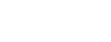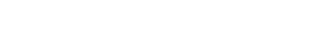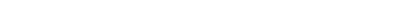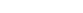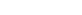—————-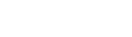I used the reduction method: A+A, –B+B and 0+1. To find the value of B we are going to use substitution: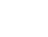After substitution we have: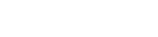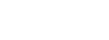We can now plug in A and B: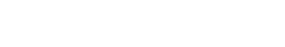As last thing, let’s multiply by u²: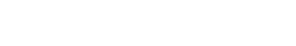Now I can tell you a faster method for decomposing fractions; it actually comes from the one I have just shown you, this is why I didn’t explain it before.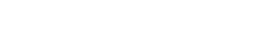Then you write it as we did before:The value of A is given by the reciprocal of 1+u, where u is the value you need in order to make 1-u equal to 0, which is 1 (1-1=0).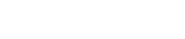And the same is for B, but the other way around. To remember this, think that to find A you need the denominator of B and for B the denominator of A. 1+u=0 when u=-1. So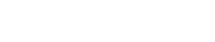As we saw before, these results are correct.

Back to the integral: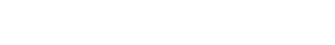Let’s apply linearity: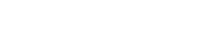Now we can let v=1-u for the first integral and t=1+u for the second.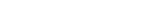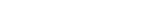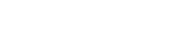Since v=1-u, u=1-v and u²=(1-v)²; also, t=1+u, u=t-1 and u²=(t-1)².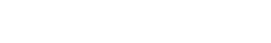Now everything is very basic: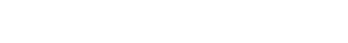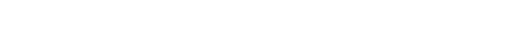Whoah! So many integrals! But they are super easy!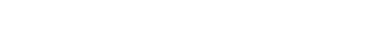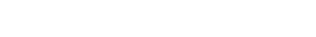We are finally ready to undo all of the substitutions! Remember: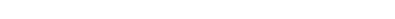This is going to be the result of the integral of sin²(x)/cos(x):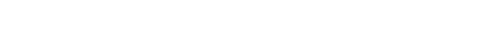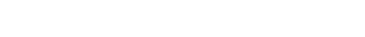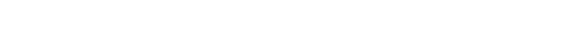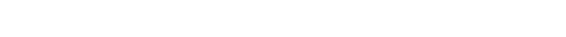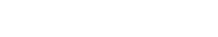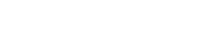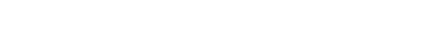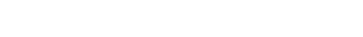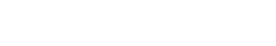Therefore: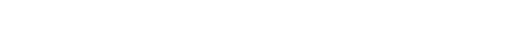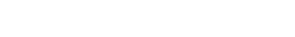We could stop here, but we can do a few more things to make this nice and compact: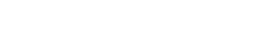We can apply the properties of logarithms: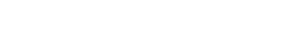At this point I noticed that if I multiply top and bottom by the square root of 1+sin(x), I get a square at the nominator and a difference of squares at the denominator, which is equal to cos²(x).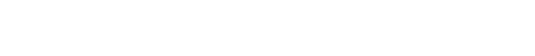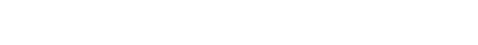We’re almost done!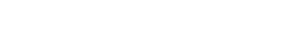You can now take a deep breath! This is the result!

Now I challenge you to find the integral of csc(x). The procedure is pretty much the same. Leave the answer in the comments!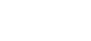I really hope you found this helpful and that everything was clear! If you have any questions, don’t hesitate to ask in the comments, I will be happy to reply! If you liked this post give it a like and subscribe for more, it would mean a lot to me!

Join 31 other subscribers.